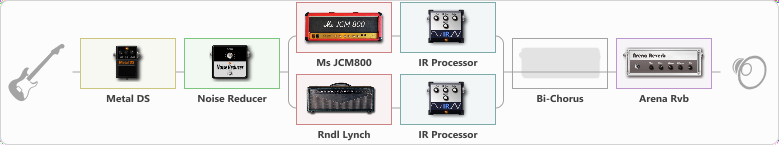# JCM 800 + RANDALL LYNCH + MESA 4x12 @ sm57 v30

Discussion in 'ToneLib-GFX presets' started by Summers, Feb 25, 2023.

1. JCM 800 + RANDALL LYNCH + MESA 4x12 @ sm57 v30

Preset name: JCM 800 + RANDALL LYNCH + MESA 4x12 @ sm57 v30

Bridge Pickup. You can replace METAL DS with TS.

Effects chain:Effect: "Metal DS" (Overdrive / Distortion), active - "yes"
{
"Dist" = 100
"Bass" = 66
"Middle" = 60
"Treble" = 46
"Level" = 57
}

Effect: "Noise Reducer" (Dynamics / Filter), active - "yes"
{
"Sens" = 89
"Mode" = Soft
}

Effect: "Splitter" (Dynamics / Filter)
{
"A-Bypass" = Off
"A-Pan" = -25
"A-Level" = 63
"B-Bypass" = Off
"B-Pan" = 25
"B-Level" = 64
"Width" = 0

'A' branch:
{

Effect: "Ms JCM800" (Amp simulators), active - "yes"
{
"Gain" = 81
"Bass" = 50
"Middle" = 40
"Treble" = 42
"Presence" = 37
"Master" = 69
"Level (dB)" = 0
}

Effect: "IR Processor" (Cabinets), active - "yes"
{
"IR" = Brohymn-Mesa-4x12-SM57-V30-5
"Low Cut (Hz)" = 0
"Hi Cut (kHz)" = 20.0
"Mix" = 100
"Level (dB)" = 0
}
}
'B' branch:
{

Effect: "Rndl Lynch" (Amp simulators), active - "yes"
{
"Gain" = 74
"Bass" = 73
"Middle" = 49
"Treble" = 49
"Presence" = 100
"Master" = 100
"Level (dB)" = 3
}

Effect: "IR Processor" (Cabinets), active - "yes"
{
"IR" = Brohymn-Mesa-4x12-SM57-V30-5
"Low Cut (Hz)" = 0
"Hi Cut (kHz)" = 20.0
"Mix" = 100
"Level (dB)" = 0
}
}
}

Effect: "Bi-Chorus" (Modulation / Sfx), active - "no"
{
"Speed A" = 3.2
"Speed B" = 4.0
"Depth" = 72
"Reso" = 0
"Mode" = Parallel
"Mix" = 56
}

Effect: "Arena Rvb" (Reverberation), active - "yes"
{
"Time" = 7.3
"PreDelay" = 23
"LoDamp" = 18
"HiDamp" = 21
"Mix" = 43
}

Note: You will need to download and install the ToneLib-GFX software to use the preset.

File size:
15.3 KB
Views:
3,125